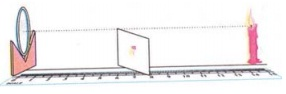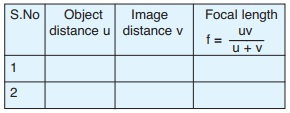## 10th Class Physical Sciences – Important Bits for Public Exams of AP and TS Boards

Following are important objective multiple choice questions for 10th Class public examinations of AP and Telangana School Education Boards. The MCQs are highly scoring. Students can practice all the bits to score well in public exams. Answers are given at the end of questions. Apart from these, 1 and 2 marks questions play important role in scoring good marks in Physical sciences. All the best.

1. Internal energy of a system is …
i) Potential energy ii) Vibrational energy
iii) Rotational and linear kinetic energy
A) i is correct B) i, ii and iii are correct
C) iii is correct D) i and iii are correct

2. A teacher held a pencil close to a spherical mirror and asked four students P, Q, R and S to predict the nature of the mirror with the help of the image formed in the mirror. The image was erect and enlarged
P – Concave in nature, Q – Convex in nature
R – Plane mirror, S – Plano-Concave mirror
A) P B) Q C) R D) S

3. Mirage is ….
X) An optical illusion
Y) Due to denser upper layer and rarer lower layer in the air on the road.
A) X and Y are true
B) X is true and Y is false
C) X is false and Y is true
D) Both X and Y are false

4. The magnetic force on a charge moving parallel to a magnetic field is ….
A) Bqv B) ∞ C) Bqv sin θ D) 0

5. A thick wire has a …… resistance than a thin wire.
A) less B) equal C) more D) high

6. Match the following:
I) Total internal reflection ( ) P) Snell’s law
II) Refraction ( ) Q) Dish antenna
III) Reflection ( ) R) Optical fibres

A) I-P, II-Q, III-R B) I-R, II-P, III-Q
C) I-Q, II-R, III-P D) I-Q, II-P, III-R

7. An atom of an element has 12 protons. Then what is the position of the element in the periodic table
A) 3rd period 2nd group
B) 2nd period 2nd group
C) 2nd period 3rd group
D) 3rd period 3rd group

8. This is suitable for use in heating elements of electric stove ….
A) Nickel B) Nichrome
C) Copper D) Iron

9. The maximum number of carbon atoms in a hydrocarbon to show isomerism
A) 2 B) 3 C) 1 D) 4

10. If 2 ml of acetic acid was added slowly in drops to 5 ml of water then we will notice
A) The acid forms a separate layer on the top of water
B) Water forms a separate layer on the top of the acid
C) Formation of a clear and homogeneous solution
D) Formation of a pink and clear solution

11. When two convex lenses are put in contact, their resultant focal length…
A) increases B) decreases
C) remains constant D) is Zero

1-B
2-A
3-A
4-D
5-A
6-B
7-A
8-B
9-D
10-C
11-B.

## 10th Class Physical Sciences – Imp Questions of 4 Marks and Bits from Reflection of Light at Curved Surfaces

Following are the important 4 Marks Questions for SSC / 10th Class public exams for AP and Telangana students as per CCE pattern of syllabus. These questions are from the chapter of Physical Sciences, Reflection of Light at Curved Surfaces:

Q: List the materials required for conducting an experiment to find the focal length of a concave mirror. Explain the experimental process also.
A: Materials required: 1) Concave mirror 2) Candle 3) Paper screen 4) v-stand 5) Meter Scale

Procedure:Place the concave mirror on v-stand, a candle, paper screen and meter scale as shown in the figure.

Put the candle at different distances from the mirror along the scale and by moving the screen find the position where the sharp
image is formed on the paper screen.

In each case note the distance (u) of the candle from the mirror and the respective screen distance (v) from the mirror

Tabulate the observations.Find the average of focal lengths (f) obtained.
This average value of focal length (f) is the focal length of the concave mirror.

Part – B

1. An object is placed at 5 cm distance in front of a concave mirror of 6 cm focal length, then the nature of the image is
A) Real and inverted B) Virtual and erect
C) Virtual and inverted D) Real and erect

2. Sita is searching for light of parallel beam.
This source can help her….
A) Torch B) Candle C) Sun D) Sodium vapour lamp

3. The shape of solar cooker is
A) Concave B) Plane C) Ellipsoid D) Convex